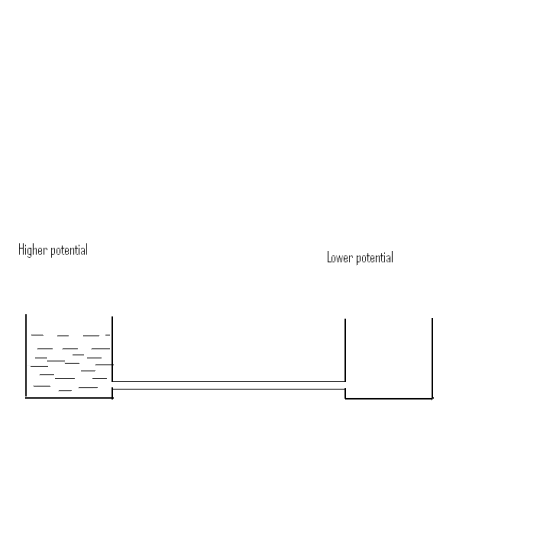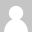# Basics Of Electricity

2009-05-29

Now lets see some basic concepts involved in Electrical Engineering which includes Current,Voltage,Potential Difference, Emf and Ohms Law.

We often use the term current in our day to day life.Lets see the definition of current

Current:

Current is defined as the flow of electric charge between two points of a section (or) a conductor.

We very well know the unit of charge. Suppose if u don't,The unit of charge is COULOMB.

The unit of current is Ampere.

From above two statements we can conclude Ampere= Coulomb/second.

Now lets define what is ONE AMPERE:

One ampere current is defined as the rate of flow of one coulomb of electric charge through a section for one second.

Voltage:

A voltage can be defined as the potential difference that exists between two points.

Voltage can also be defined in terms of the energy required to further charge the body (or) Potential Energy per unit charge on the body.

Therefore we can define ONE VOLT as:

The body is said to have a potential of one volt if 1 joule of work is done to put 1Coulomb of charge on a charged body.

Potential Difference:
If two water tanks having water at different levels are connected together using a pipe,then water will flow from higher level to lower level,till the water level in two tanks are equal. When the water levels in tank (or) beaker is equal,all flow will stop.

Now same concept is applied to electricity. If two points in a electric circuit are at different electric potential and if the two points are connected through a wire or a conductor,current will flow from higher potential to lower potential and try to equalize the potential between the points.Electro Motive Force:

Electromotive force of a device is defined as the energy per unit charge provided by device to the circuit.

Electromotive force is also defined as the difference in electric potential between the terminals of source of electricity(eg:battery from which no current is supplied to circuit)

Suppose If Q is the unit charge provided by the device and W is the energy then

EMF=W/Q. Its unit is Volt (or) joules/coulomb.

OHM'S Law:

According to Ohms law, the potential difference that exist between two points of a conductor is directly proportional to the current through the same conductor,provided the temperature remains constant.

Ohms law: V directly proportional to I at constant temperature.

V=IR where I-> current through the conductor
R-> Resistance of the conductor

The greatest disadvantage of ohms law is, It holds good for constant temperature conditions. And it is not applicable to varying temperature conditions.

•Unknown

can u pls explain clearly what is voltage, potential difference, and electro motive force?
can you give everyday life example where we have two bodies with different potential difference?
or you mean the 2 bodies just connected by a wire... or be placed in a closed circuit...assume me as body 'A' and you as body 'B', and then if we are connected by a wire....does potential flow? hope you know that human body also has resistance...which means we conduct electricity..proof: we get shock ....

•Unknown

hello sriram,
I changed my definition of potential difference..sorry for posting it wrong.Actually potential difference is the difference in potential between two points in a electric circuit. I dont know whether there is any every day example...if u know please share it with me..

•brandMARIO

Hey... I'm taking part in the My Demand Contest. Ever thought of converting your "Pee to Petrol"??

http://www.indiblogger.in/indipost.php?post=30610

Please do vote for this. I bet you'll like it ;-) And do share it with others if possible.
Thank you :-)